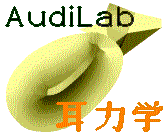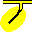#HandJ: Hooke-and-Jeeves minimization

## Introduction

`handj()` is a Fortran subroutine that minimizes a given function using the algorithm of Hooke & Jeeves (1961).

`handj_ext` is a programme that uses `handj()` with an externally computed function.

## Usage

Have available a programme or script that can also be invoked from the command line and that evaluates the function to be minimized. The programme or script should read the function parameters from a single-line text file in the directory from which it is run, and should write the resulting function value into a single-line text file in the same directory.

Sample function script for Octave:
``` p = load ('handj_ext_param.txt'); nparams = length(p); f = 100*(p(2) - p(1)^2)^2 + (1.-p(1))^2; save -ascii handj_ext_result.txt f; ```

Run `handj_ext` from the command line. You will be asked for

1. the command with which to invoke the function-evaluation programme or script (default = `octave -q handj_ext.m > /dev/null`, where the `-q` just tells Octave not to print the usual messages when it starts)
2. the name of the file from which it reads the function parameters (default = `handj_ext_param.txt`)
3. the name of the file into which it write the function value (default = `handj_ext_result.txt`)

You will then be asked for

1. the number of parameters that the function takes
2. the initial parameter values
3. the starting value for the step size Δ
4. the minimum step size δ
The `handj()` subroutine will then be called repeatedly until Δ < δ. Note that a single Δ applies to all of the function's parameters, so the function should be defined so that all of its parameters are of the same order of magnitude.

During the search, some progress messages will be displayed. Pressing any key on the keyboard will terminate the search.

Once the search has finished, the final parameter values will be printed. A log will be dumped to the file `handj_ext.log`. For each function evaluation the log will contain the sequence number; the function value; a code that indicates what mode the algorithm was in when it called the function; and the values of the parameters. The mode codes are the following:

• S: start
• E and e: explore (different phases of the algorithm)
• P: make a pattern move

The programme will then offer to graphically display the evolution of two of the parameters. You will be asked which parameters to plot. Type control-Z if you do not want a graphical display.AudiLab home page
R. Funnell